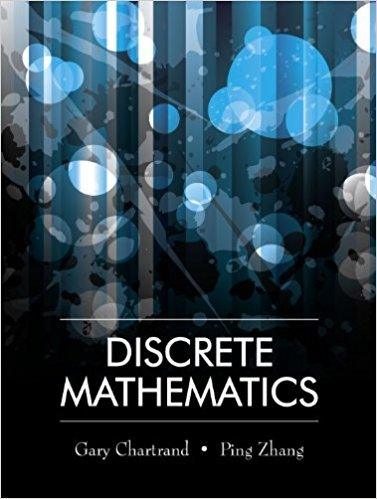×
Get Full Access to Discrete Mathematics - 1 Edition - Chapter 3.4 - Problem 1
Get Full Access to Discrete Mathematics - 1 Edition - Chapter 3.4 - Problem 1

×

# Prove that if n is an integer, then n2 3n + 5 is oddISBN: 9781577667308 385

## Solution for problem 1 Chapter 3.4

Discrete Mathematics | 1st Edition

• Textbook Solutions
• 2901 Step-by-step solutions solved by professors and subject experts
• Get 24/7 help from StudySoup virtual teaching assistantsDiscrete Mathematics | 1st Edition

4 5 1 332 Reviews
17
1
Problem 1

Prove that if n is an integer, then n2 3n + 5 is odd.

Step-by-Step Solution:
Step 1 of 3

Section 2.2­3.2: 1) Radicals and Power functions reflect over the line y=x; Radicals start increasing at a faster pace and then start to even out, where power functions start increasing slowly then more rapidly. 2) Smaller Inputs have larger Radicals, where larger inputs have larger power functions. a) The product, quotient, and power rule of Radicals do not work if the radicals have more than one term. 3) If the domain or range of the radical functions are (negative infinity, negative infinity) it stays the same. 4) For polynomial and Rational power inequalities/equations you need to factor the equation down until you find the zeros. 5) The domain of rational functions is found by breaking t

Step 2 of 3

Step 3 of 3

##### ISBN: 9781577667308

Unlock Textbook Solution